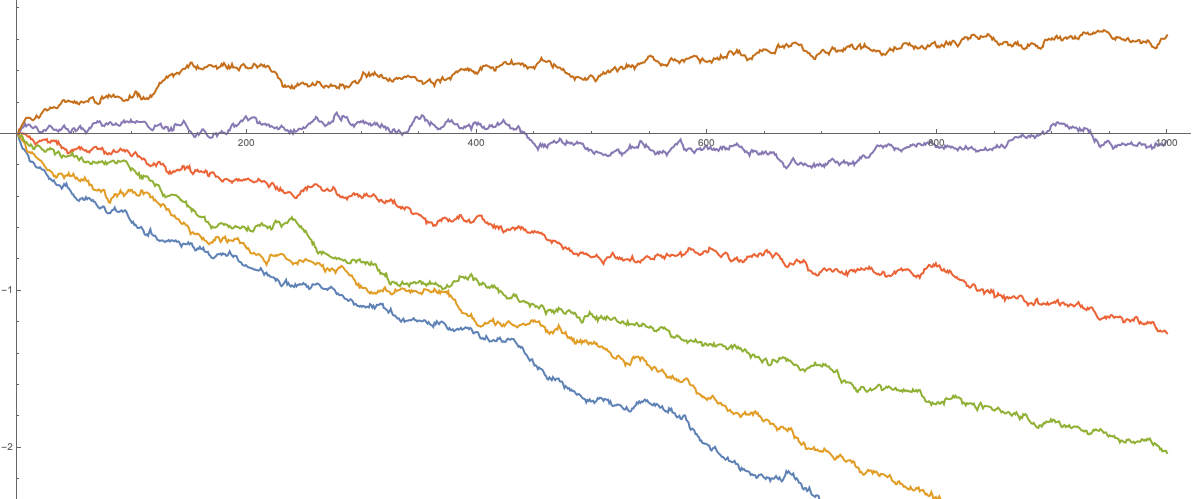# Parameter symmetry in perturbed GUE corners process and reflected drifted Brownian motions

### 2019/12/17

(with Mikhail Tikhonov)
arXiv:1912.08671 [math.PR]

PDF

The perturbed GUE corners ensemble is the joint distribution of eigenvalues of all principal submatrices of a matrix $G+\mathrm{diag}(\mathbf{a})$, where $G$ is the random matrix from the Gaussian Unitary Ensemble (GUE), and $\mathrm{diag}(\mathbf{a})$ is a fixed diagonal matrix. We introduce Markov transitions based on exponential jumps of eigenvalues, and show that their successive application is equivalent in distribution to a deterministic shift of the matrix. This result also leads to a new distributional symmetry for a family of reflected Brownian motions with drifts coming from an arithmetic progression.

The construction we present may be viewed as a random matrix analogue of the recent results of the first author and Axel Saenz.Perturbed Dyson Brownian motion - eigenvalue dynamics of a 6x6 matrix of Brownian motions, with no drift off the diagonal, and an arithmetic progression of drifts on the diagonal